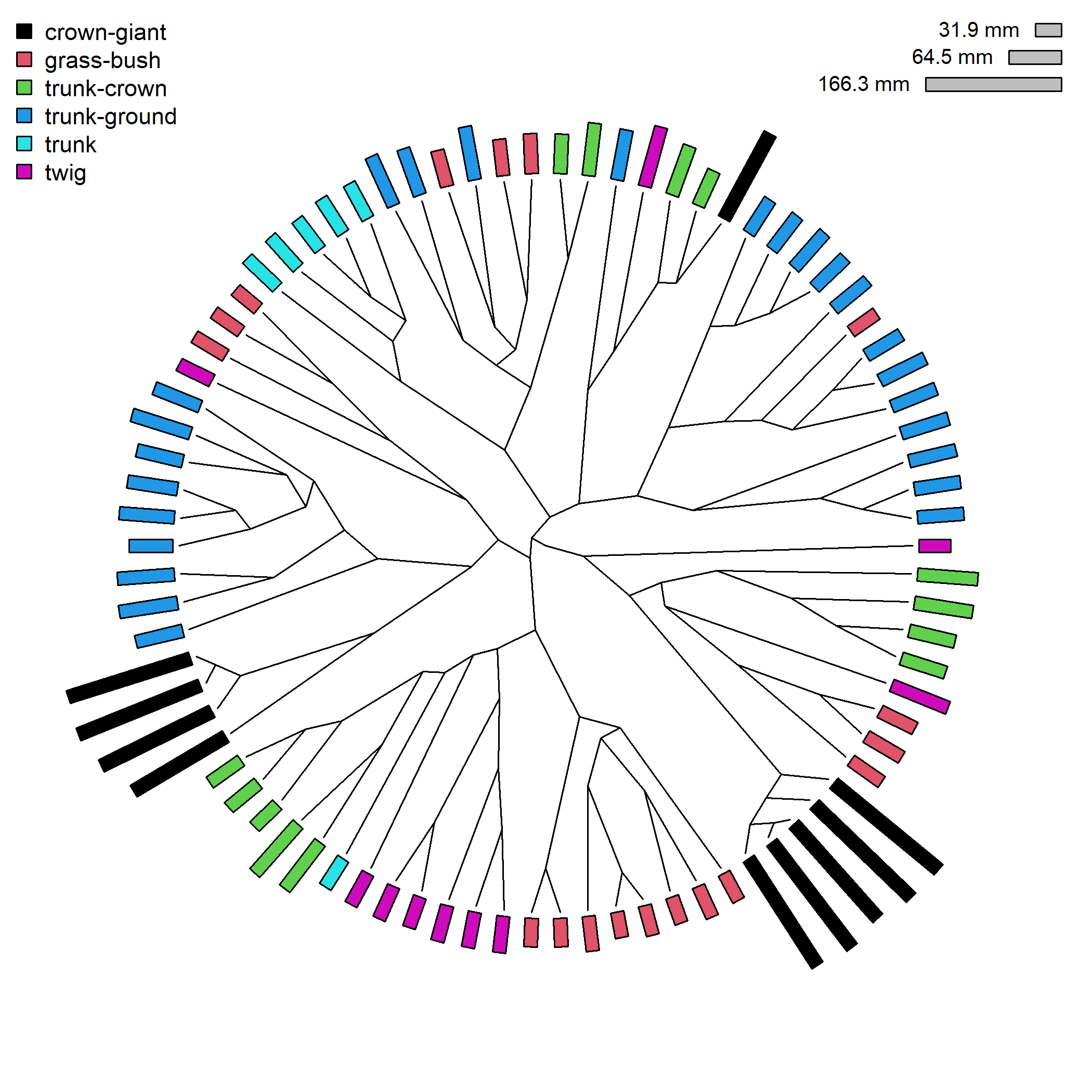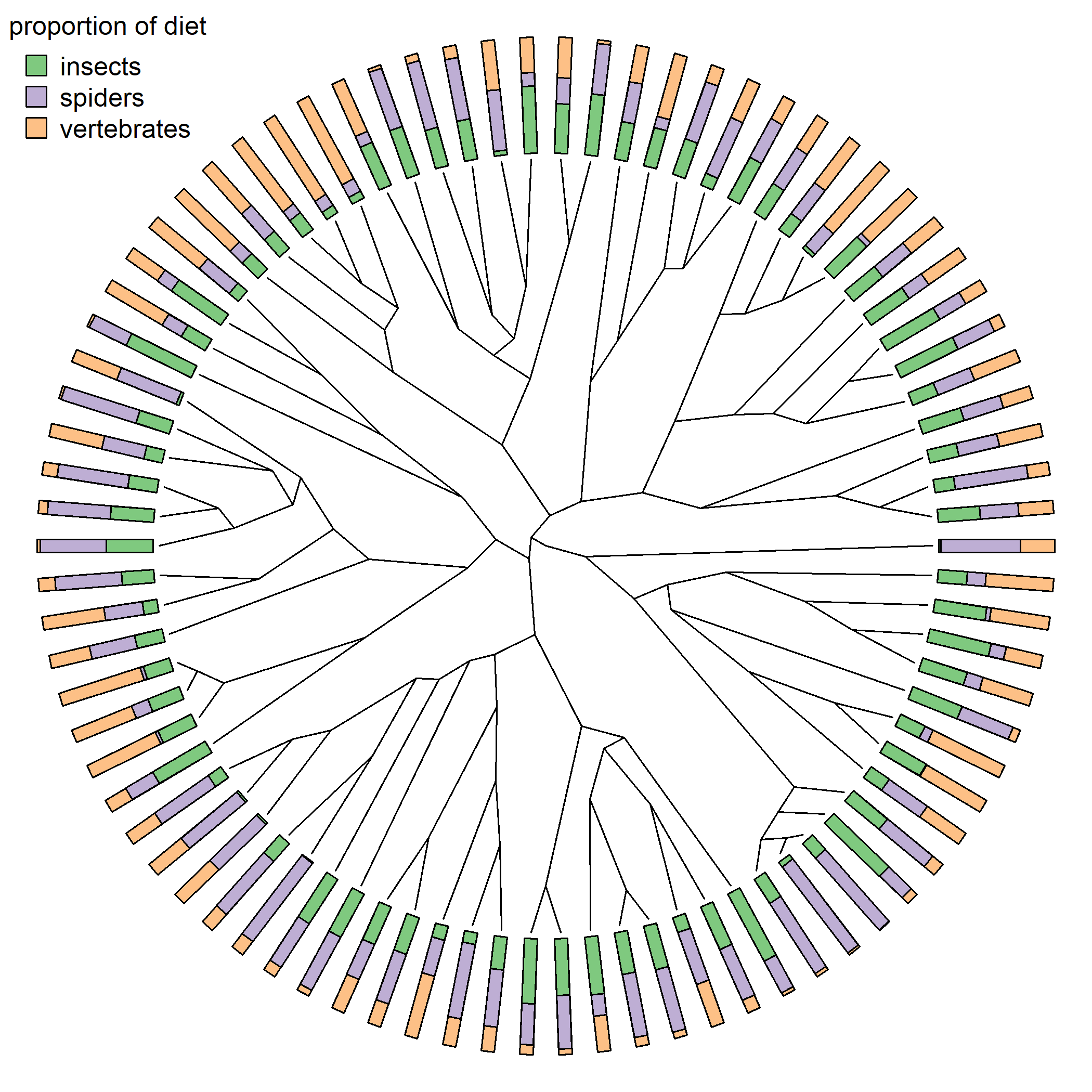## Sunday, January 22, 2023

### `plotTree.wBars` in "radial" style

Several months ago, I posted a relatively straightforward method to graph “radial” style phylogeny.

A radial style tree graphs the phylogeny in a circular style in which distance from the origin (i.e., the center of the circle) corresponds to the height above the root of each node or tip.

The difference between a “fan” and a “radial” tree is that in the case of a radial tree, edges are plotted in a slanted style – rather than along radii of the (hypothetical) circle that has its center at the origin.

Recently, a phytools blog reader asked if the method could “be somehow combined with `plotTree.wBars` and `arc.cladelabels`?

The answer is, of course, “sure.” In this post, I just start with `plotTree.wBars`. For my first example I’ll use the tree `anoletree` and body size of Anolis lizards in `anole.data`. For fun, I’m going to color the bars using the “ecomorph” of each Anolis species in the tree. I’ll also include a legend showing both the color palette of the discrete character at the tips, and the scale of the bar lengths. (The latter really ought to be included by default in this function. Perhaps I’ll add it to a future version!)

``````library(phytools)
data(anoletree)
## get ecomorph state
ecomorph<-as.factor(getStates(anoletree,"tips"))
cols<-setNames(palette()[1:length(levels(ecomorph))],
levels(ecomorph))
cols<-setNames(cols[ecomorph],names(ecomorph))
## convert anoletree to simple "phylo" object
anoletree<-as.phylo(anoletree)
data(anole.data)
svl<-setNames(exp(anole.data\$SVL),rownames(anole.data))
plotTree.wBars(anoletree,svl,type="fan",color="transparent",
col=cols)
pp<-get("last_plot.phylo",envir=.PlotPhyloEnv)
for(i in 1:nrow(pp\$edge))
lines(pp\$xx[pp\$edge[i,]],pp\$yy[pp\$edge[i,]])
legend("topleft",c("crown-giant","grass-bush","trunk-crown",
"trunk-ground","trunk","twig"),pch=22,
pt.bg=palette()[1:length(levels(ecomorph))],pt.cex=1.4,
cex=0.9,bty="n")
scale<-0.3*max(nodeHeights(anoletree))/diff(range(svl))
w<-(par()\$usr-par()\$usr)/(max(c(max(scale*svl)/
max(nodeHeights(anoletree)),1))*Ntip(anoletree))
xx<-0.95*par()\$usr
yy<-0.95*par()\$usr
polygon(c(xx,xx-scale*min(svl),xx-scale*min(svl),xx),
c(yy-w/2,yy-w/2,yy+w/2,yy+w/2),col="grey")
text(xx-scale*min(svl),yy,paste(round(min(svl),1),"mm"),
pos=2,cex=0.8)
xx<-0.95*par()\$usr
yy<-0.9*par()\$usr
polygon(c(xx,xx-scale*mean(svl),xx-scale*mean(svl),xx),
c(yy-w/2,yy-w/2,yy+w/2,yy+w/2),col="grey")
text(xx-scale*mean(svl),yy,paste(round(mean(svl),1),"mm"),
pos=2,cex=0.8)
xx<-0.95*par()\$usr
yy<-0.85*par()\$usr
polygon(c(xx,xx-scale*max(svl),xx-scale*max(svl),xx),
c(yy-w/2,yy-w/2,yy+w/2,yy+w/2),col="grey")
text(xx-scale*max(svl),yy,paste(round(max(svl),1),"mm"),
pos=2,cex=0.8)
``````To close this up, I’ll also show how to combine this visualization with a previous hack illustraing how to use `plotTree.wBars` to show stacked bars.

I’ll start by simulating data that I can use for the visualization – in this case, I’ll generate three different characters that sum to 1.0. Let’s just imagine these are proportions of the diet in three different categories.

``````X<-fastBM(anoletree,nsim=3,bounds=c(0,Inf))
X<-t(apply(X,1,function(x) x<-x/sum(x)))
colnames(X)<-c("insects","spiders","vertebrates")
``````

Now let’s create our plot. (For an explainer of how this works – see my previous post!)

``````Y<-t(apply(X,1,cumsum))
Y<-t(apply(X,1,cumsum))
library(RColorBrewer)
cols<-brewer.pal(n=ncol(Y),"Accent")
scale<-0.3*max(nodeHeights(anoletree))
plotTree.wBars(anoletree,Y[,ncol(Y)],type="fan",
color="transparent",scale=scale,col=cols[ncol(Y)])
pp<-get("last_plot.phylo",envir=.PlotPhyloEnv)
for(i in 1:nrow(pp\$edge))
lines(pp\$xx[pp\$edge[i,]],pp\$yy[pp\$edge[i,]])
for(i in (ncol(Y)-1):1){
plotTree.wBars(anoletree,Y[,i],type="fan",scale=scale,
col=cols[i])
}
legend(x="topleft",legend=colnames(Y),pch=22,
pt.cex=2,pt.bg=cols,bty="n",horiz=FALSE,
title="proportion of diet")
``````Nice!